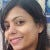# List Comprehension

`a=[1,2,3]b=[]for i in a: k=i*i b.append(k)print(b)[1, 4, 9]`
`a=[1,2,3]k=[i*i for i in a]print(k)[1, 4, 9]`
`n=[21,102,56,33,99,101,36,33]k=[]for i in n: if i%2!=0:  k.append(i)print(k)[21, 33, 99, 101, 33]`
`n=[21,102,56,33,99,101,36,33]k=[i for i in n if i%2!=0]print(k)[21, 33, 99, 101, 33]`

# Encoding Categorical Variables

## Label Encoding and One Hot Encoding